Hostname: page-component-797576ffbb-pxgks Total loading time: 0 Render date: 2023-12-10T05:54:15.222Z Has data issue: false Feature Flags: { "corePageComponentGetUserInfoFromSharedSession": true, "coreDisableEcommerce": false, "useRatesEcommerce": true } hasContentIssue false

# Maximal Weight Composition Factors for Weyl Modules

Published online by Cambridge University Press:  20 November 2018

## Abstract

Core share and HTML view are not possible as this article does not have html content. However, as you have access to this content, a full PDF is available via the ‘Save PDF’ action button.

Fix an irreducible (finite) root system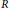$R$ and a choice of positive roots. For any algebraically closed field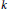$k$ consider the almost simple, simply connected algebraic group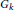${{G}_{k}}$ over$k$ with root system$k$. One associates with any dominant weight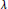$\lambda$ for$R$ two${{G}_{k}}$-modules with highest weight$\lambda$, the Weyl module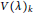$V{{(\lambda )}_{k}}$ and its simple quotient$V{{(\lambda )}_{k}}$. Let$\lambda$ and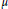$\mu$ be dominant weights with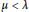$\mu <\lambda$ such that$\mu$ is maximal with this property. Garibaldi, Guralnick, and Nakano have asked under which condition there exists$k$ such that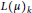$L{{(\mu )}_{k}}$ is a composition factor of$V{{(\lambda )}_{k}}$, and they exhibit an example in type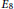${{E}_{8}}$ where this is not the case. The purpose of this paper is to to show that their example is the only one. It contains two proofs for this fact: one that uses a classiffication of the possible pairs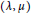$(\lambda ,\mu )$, and another that relies only on the classiûcation of root systems.

## Keywords

Type
Research Article
Information
Canadian Mathematical Bulletin , 01 December 2017 , pp. 762 - 773